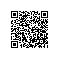# JavaScript总结

JavaScript 是世界上最流行的脚本语言。
JavaScript 是属于 web 的语言，它适用于 PC、笔记本电脑、平板电脑和移动电话。
JavaScript 被设计为向 HTML 页面增加交互性。

1.JavaScript：写入 HTML 输出

document.write(“h1 This is a heading /h1”);
document.write(“p This is a paragraph /p”);
2.HTML 中的脚本必须位于 script 与 /script 标签之间。

script 标签

script 和 script 会告诉 JavaScript 在何处开始和结束。
script 和 script之间的代码行包含了 JavaScript：
script
script
bod 中的 JavaScript

!DOCTYPE html
html
body

script
document.write(“h1 This is a heading /h1”);
document.write(“p This is a paragraph /p”);
/script
body
html
3.JavaScript 通常用于操作 HTML 元素。

!DOCTYPE html
html
body
h1我的第一张网页/h1
p id=”demo”我的第一个段落/p
script
document.getElementById(“demo”).innerHTML=”我的第一段 JavaScript”;
/script
/body
/html
4.JavaScript 注释
body

h1 id=”myH1”/h1

p id=”myP” /p

script
// 输出标题：
document.getElementById(“myH1”).innerHTML=”Welcome to my Homepage”;
// 输出段落：
document.getElementById(“myP”).innerHTML=”This is my first paragraph.”;

p b注释：/b注释不会被执行。/p

/body
/html
5.JavaScript 变量
var x=2;
var y=3;
var z=x+y;
var值是什么就是什么数据类型
var pi=3.14;
var name=”Bill Gates”;
6.一条语句，多个变量
var name=”Gates”, age=56, job=”CEO”;

var name=”Gates”,
age=56,
job=”CEO”;

var carname=”Volvo”;
var carname;

body
p假设 y=5，计算 x=y+2，并显示结果。/p
button onclick=”myFunction()”点击这里/button
p id=”demo”/p
script
function myFunction()
{
var y=5;
var x=y+2;
var demoP=document.getElementById(“demo”)
demoP.innerHTML=”x=” + x;
}
/script
/body
7.JavaScript 数据类型

JavaScript 拥有动态类型
JavaScript 拥有动态类型。这意味着相同的变量可用作不同的类型：

var x // x 为 undefined
var x = 6; // x 为数字
var x = “Bill”; // x 为字符串
JavaScript 字符串

var carname=”Bill Gates”;
var carname=’Bill Gates’;
8.JavaScript 对象
JavaScript 中的所有事物都是对象：字符串、数字、数组、日期，等等。

car.name=Fiat
car.model=500
car.weight=850kg
car.color=white

car.start()
car.drive()
car.brake()

objectName.propertyName

var message=”Hello World!”;
var x=message.length;
9.script
\$(function(){
{
}
})
/script
body
button onclick=”myFunction()”点击这里/button
/body
/html
10JavaScript 运算符

y=5;
z=2;
x=y+z;
+ 加 x=y+2 x=7
- 减 x=y-2 x=3
* 乘 x=y*2 x=10
/ 除 x=y/2 x=2.5
% 求余数 (保留整数) x=y%2 x=1
++ 累加 x=++y x=6
– 递减 x=–y x=4
8.JavaScript 比较和逻辑运算符

== 等于 x==8 为 false
=== 全等（值和类型） x===5 为 true；x===”5” 为 false
!= 不等于 x!=8 为 true

< 小于 x<8 为 true
= 大于或等于 x>=8 为 false
<= 小于或等于 x<=8 为 true
9.JavaScript If…Else 语句
if 语句 - 只有当指定条件为 true 时，使用该语句来执行代码
if…else 语句 - 当条件为 true 时执行代码，当条件为 false 时执行其他代码
if…else if….else 语句 - 使用该语句来选择多个代码块之一来执行
switch 语句 - 使用该语句来选择多个代码块之一来执行

if (time<20)
{
x=”Good day”;
}

if (time<20)
{
x=”Good day”;
}
else
{
x=”Good evening”;
}

if (time<10)
{
x=”Good morning”;
}
else if (time<20)
{
x=”Good day”;
}
else
{
x=”Good evening”;
}

if (time<10)
{
x=”Good morning”;
}
else if (time<20)
{
x=”Good day”;
}
else
{
x=”Good evening”;
}
10 JavaScript For 循环
document.write(cars + “br”);
document.write(cars + “br”);
document.write(cars + “br”);
document.write(cars + “br”);
document.write(cars + “br”);
document.write(cars + “br”);

for (var i=0;i《cars .length;i++)
{
document.write(cars[i] + “br”);
}
For/In 循环
JavaScript for/in 语句循环遍历对象的属性：

var person={fname:”John”,lname:”Doe”,age:25};
for (x in person)
{
txt=txt + person[x];
}
11.JavaScript While 循环

while (i<5)
{
x=x + “The number is ” + i + “
”;
i++;
}
break 语句用于跳出循环。
continue 用于跳过循环中的一个迭代。

for (i=0;i<10;i++)
{
if (i==3) break;
x=x + “The number is ” + i + “
”;
}
12.
JavaScript 错误 - Throw、Try 和 Catch
JS Break
JS 验证
try 语句测试代码块的错误。
catch 语句处理错误。
13.JavaScript 表单验证

{
with (field)
{
if (value==null||value==”“)
else {return true}
}
}

html
script type=”text/javascript”

{
with (field)
{
if (value==null||value==”“)
else {return true}
}
}

function validate_form(thisform)
{
with (thisform)
{
if (validate_required(email,”Email must be filled out!”)==false)
{email.focus();return false}
}
}
/script

body
form action=”submitpage.htm” onsubmit=”return validate_form(this)” method=”post”
Email: input type=”text” name=”email” size=”30”
input type=”submit” value=”Submit”
/form
/body
/html
14.JavaScript 能够改变页面中的所有 HTML 元素
JavaScript 能够改变页面中的所有 HTML 属性
JavaScript 能够改变页面中的所有 CSS 样式
JavaScript 能够对页面中的所有事件做出反应

15.HTML DOM 允许 JavaScript 改变 HTML 元素的内容。

document.getElementById(id).innerHTML=new HTML

html
body

p id=”p1”>Hello World! /p

script
document.getElementById(“p1”).innerHTML=”New text!”;
/script

/body
/html
16改变 HTML 属性

document.getElementById(id).attribute=new value

!DOCTYPE html
html
body

img id=”image” src=”smiley.gif”

script
document.getElementById(“image”).src=”landscape.jpg”;
/script

/body
/html使用钉钉扫一扫加入圈子
+ 订阅